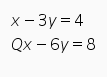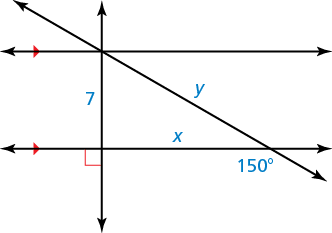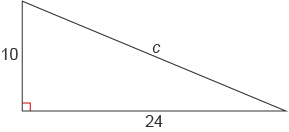# Find the value of Q in the following system so that the solution to the system is {(x,y): x-3y=4} ?$Q = 2$

#### Explanation:

just multiply the entire first function by 2

therefore,
$\left(x - 3 y = 4\right) \cdot 2$
=

therefore,
$= 2 x - 6 y = 8$
=

this is the same as the second function and in it, the coefficient of x is 2, therefore $Q = 2$

##### Add Answer of: Find the value of Q in the following system so that the solution to the system is {(x,y): x-3y=4} ?
Similar Homework Help Questions

• ### What are the values of x and y?What is the answer in simplest form?• ### What is the value of c?Need Online Homework Help?